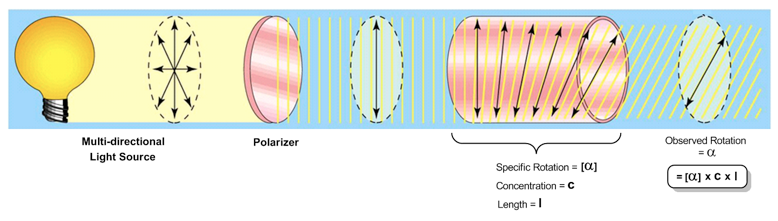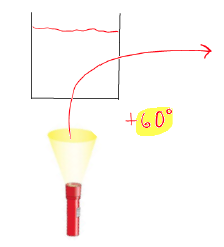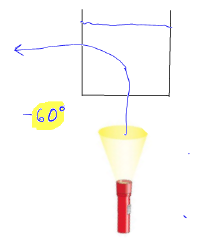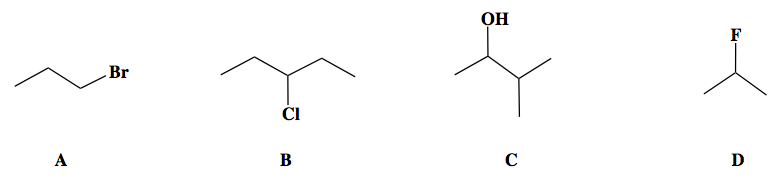Clutch Prep is now a part of Pearson
Ch.20 - Organic ChemistryWorksheetSee all chapters

# Optical Isomers

See all sections
Sections
Introduction to Organic Chemistry
Structural Formula
Chirality
Optical Isomers
Hydrocarbon
The Alkyl Group
Naming Alkanes
Naming Alkenes
Naming Alkynes
Alkane Reactions
Alkenes and Alkynes
Benzene Reactions
Functional Groups
Alcohol Reactions
Carboxylic Acid Derivative Reactions

Optiical activity deals with the ability of a solution to rotate plane polarized light.

###### Chirality and Optical Activity

Concept #1: Polarimeter

Transcript

Hey guys, in this new video, we're going to take a look at a concept known as optical activity.
We're going to say here we recently learned that a chiral molecule is basically a molecule that contains a carbon that is connected to four different things. We're going to say one key feature of chiral molecules is that they rotate plane polarized light.
What we need to realize here is that we have a light source, so a light bulb or a flashlight, anything hat can omit light. What normally happens with regular light is that it oscillates. Basically, the light goes in all directions. Then what's the effect of this? If we were to shine that light through some type of solution, the light would go through the solution. The special thing about chiral centers is if we have a solution made up of a chiral molecule, it will actually bend the light. The light will actually bend to the left or to the right. It won't shine through the solution.
In order for us to determine how much to the left or to the right that it bends, we use what's called a polarimeter. Basically, this is the apparatus for polarimeter. What the polarimeter measures is the observed rotation alpha. This basically tells me does it bend 60 degrees to the left, which would be negative 60 degrees or does it bend 60 degrees to the right, which would be a positive 60 degrees.
We have terms that are associated with this bending of light. Here what we have is two solutions Both of them have a flashlight shining on them. Here if we say the solution – we shine the light on it, let's say it bends light clockwise. When it bends light clockwise, that means it's bending light to the right. If it bends light to the right, it's known as dextrorotatory or lower case d. If it's bending light to the right, it has a positive observed rotation. Let's say this is a positive 60 degrees that I said earlier.
Here let's say we have another solution. This solution we also basically shine a light on. But let's say it bends the light counterclockwise, which means left. Here, since it bends light to the left, it's known as levorotatory. Here it's bending light to the left, so it would have a negative observed rotation. Let's say that this is negative 60 degrees.
We're gong to say that these two solutions have basically the same degree, 60. The only thing that's different about them is which direction does it point in terms of 60 degrees. We're going to say two chiral solutions that point light in completely opposite directions are enantiomers. These two solutions represent enantiomeric solutions. One chiral compound and it's exact mirror image in the other solution. They bend light in completely opposite directions.
In fact, this is one of the key differences between enantiomers. Enantiomers have the same boiling point, same melting point, same density, same formula, same mass, same connections. The only things that are different about them are their configurations, which you're not going to learn here, you're going to learn that in organic, and their observed rotation. Enantiomers only have two things that are different about them, their configurations and their direction they bend light, either to the left or to the right.
Here the names and degrees of rotation have nothing to do with the chirality of compounds. Let's say you're able to determine the configuration of one chiral center and in that example, it bent light to the right. Or you could take a different chiral center, it could have the same exact configuration as the one you just looked at, but its observed rotation could be in the opposite direction. When you guys get to organic, you'll learn in greater detail how can we exactly determine which direction does a chiral solution bend light. You'll learn about different formulas that are involved with the polarimeter.
But for right now the key thing you need to realize from this is a chiral compound can bend light to the left or to the right. If it bends light to the right, it's called dextrorotatory, which is lower case d. If you're bending light to the right, it's called the clockwise direction or clockwise rotation and it has a positive observed rotation. If you bend light to the left, you're known as levorotatory or lower case l. if you bend light to the left, you're counterclockwise in terms of rotation, which would mean you have a negative observed degree.
Now that we know that and now that we know that chiral centers create optical activity, I want you guys to answer this following question. I ask which of the following compounds would be optically active. It deals with what we just talked about. I'll give you guys some time to do this, come back and take a look at how exactly do I approach this problem and how best to answer it.

Chiral molecules can rotate plane polarized light and the exact angle of rotation can be calculated with a polarimeterIf light rotates to the right then the chiral molecule is referred to as dextrorotatoryIf light rotates to the left then the chiral molecule is referred to as levorotatoryExample #1: Which of the following compounds would be optically active?